The Frailty Model

You can use the frailty model to model correlations between failures of the same cluster. The hazard rate for the jth individual in the ith cluster is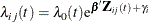where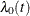is an arbitrary baseline hazard rate,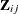is the vector of (fixed-effect) covariates,is the vector of regression coefficients, and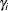is the random effect for cluster i.

Frailties are the exponential transformations of the random components, and the frailty model can be written as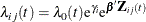The random components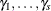(alternatively, the frailties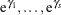) are assumed to be independent and identically distributed. Modeling is based on the random effects rather than on the frailties.

Two frailty distributions are available in PROC PHREG: gamma and lognormal. Use the DIST= option in the RANDOM statement to choose the distribution. Letbe an unknown parameter. The frailty distributions are listed in Table 67.11.

Table 67.11: Frailty Distributions

 Frailty Option Distribution Density f(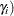Mean and Variance DIST=GAMMA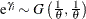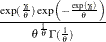E(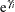)=1   V()=DIST=LOGNORMAL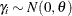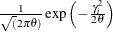E()=0    V()=The unknown parameteris a dispersion parameter. Each frailty distribution has a central tendency of 1 (the gamma frailty has a mean of 1, and the lognormal frailty has a median of 1). Thus, you can infer that individuals in cluster i with frailty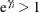(or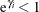) tend to fail at a faster (or slower) rate than they fail under an independence model.

PROC PHREG estimates the regression coefficients, the random effects, and the dispersion parameter. The RANDOM statement in PROC PHREG enables you to fit a shared frailty model by a penalized partial likelihood approach. If you also specify the BAYES statement, PROC PHREG performs a Bayesian analysis of the shared frailty model.

If the RANDOM statement is specified, any ASSESS, BASELINE, and OUTPUT statements are ignored. Also ignored are the COVS options in the PROC PHREG statement and the following options in the MODEL statement: BEST=, DETAILS, HIERARCHY=, INCLUDE=, NOFIT, PLCONV=, SELECTION=, SEQUENTIAL, SLENTRY=, SLSTAY=, TYPE1, and TYPE3(ALL, LR, SCORE). Profile likelihood confidence intervals for the hazard ratios are not available for the frailty model analysis.